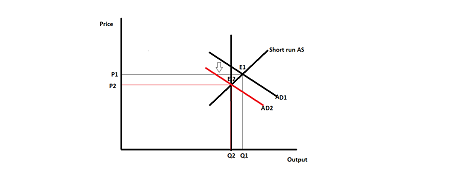# Suppose the US has real GDP above its potential, and the Federal Reserve decides to decrease the...

## Question:

Suppose the US has real GDP above its potential, and the Federal Reserve decides to decrease the money supply by selling bonds to banks on the open market. Draw the AD-AS diagram and show which curve(s) would shift in response to this policy. Be sure to label all curves, and to label the old and new equilibrium points. Then answer the following:

a. Did real GDP increase or decrease?

b. Did the price level increase or decrease?

c. Did interest rates for loans increase or decrease?

d. Which of the following types of spending likely changed in response to this policy? Select all that apply.

i. Consumption spending

ii. Investment spending

iii. Government spending

## AD-AS Model:

AD-AS model, that is aggregate demand and aggregate supply model, shows how general price level and output level is determined, graphically. The determination of price and output level takes place on the basis on relation between AD and AS.

## Answer and Explanation:

Potential output is the level of output that can be generated, by an economy, during a period of time, given all resources employed.

In short run, it may be possible that actual output level may be more than potential output level. This is known as positive output gap. To measure this gap, government may use various tools under fiscal policy. One such is selling bonds in open market operation. By selling bonds, money will flow from money market (from public) to government and the supply of money will get reduced. This will reduce consumption and investment capacity. Thus, AD curve will shift downward and new equilibrium will take place at potential output.

Consider the following graph -E1 is the equilibrium point before the open market operation and E2 is the new equilibrium after the selling of bond.

a. As you can see in the above graph, earlier output was at Q1, now it is Q2 after the operation. Thus, GDP deceases.

b. Price level also decreases from P1 to P2.

c. Due to selling of bonds in open market operation, the supply of money reduces. It increases the cost of borrowing and hence, the interest rate will increase.

d. Change in types of spending -

i. Consumption spending - It decreases because the consumption power reduces as money supply reduces.

ii. Investment spending - It also decreases because of less money supply.

iii. Government spending - It does not change.

#### Learn more about this topic:Aggregate Supply and Aggregate Demand (AS-AD) Model

from Economics 102: Macroeconomics

Chapter 7 / Lesson 3
58K